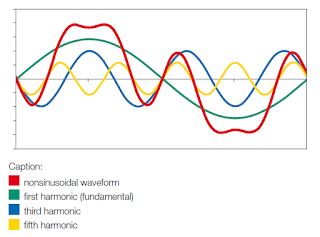### Basics of Harmonics in Electrical Systems:

Custom Search
Harmonics are essentially distortions in the electrical power systems. An electrical power system can be represented by a sinusoidal waveform which varies with time. The harmonic with frequency corresponding to the period of the original waveform is called fundamental and the harmonic with frequency equal to “n” times that of the fundamental is called harmonic component of order “n”. The presence of harmonics in an electrical system is an indication of the distortion of the voltage or current waveform and this implies such a distribution of the electric power could result in the malfunctioning of equipment and protective devices.

The harmonics are nothing less than the components of a distorted waveform and their use allows the analysis of any periodic non-sinusoidal waveform through different sinusoidal waveform components.Harmonics Distortions Waveform. Photo Credit: ABB

Causes of Harmonics in Electrical Systems
Harmonics are generated by nonlinear loads. When we apply a sinusoidal voltage to a load of this type, we shall obtain a current with non-sinusoidal waveform.

The main equipment generating harmonics are:
1. personal computer
2. fluorescent lamps
3. static converters
4. continuity groups
5. variable speed drives
6. welders.
7. Transformers (mostly third harmonics which becomes insignificant with increasing loading of the transformer).

In general, waveform distortion is due to the presence, inside of these equipment, of bridge rectifiers, whose semiconductor devices carry the current only for a fraction of the whole period, thus originating discontinuous curves with the consequent introduction of numerous harmonics.

Effects of Harmonics in Electrical Systems
The effects of harmonics can be felt in both the current and voltage.
The main problems caused by harmonic currents are:
2) increase of losses in the transformers
3) increase of skin effect.

The main effects of the harmonics voltages are:
4) voltage distortion
5) disturbances in the torque of induction motors (since Torque is proportional to supply voltage)

Total Harmonic Distortion (THD) in an Electrical System
If the rms values of the harmonic components are known, the total rms value can be easily calculated by the following formula:

Total Harmonic Distortion (THD)
The total harmonic distortion is defined as:

The harmonic distortion ratio is a very important parameter, which gives information about the harmonic content of the voltage and current waveforms and about the necessary measures to be taken should these values be high.

For THDi < 10% and THDu < 5%, the harmonic content is considered negligible and such as not to require any provisions